# The Fibonacci System

Sizing your bets according to the fibonacci sequence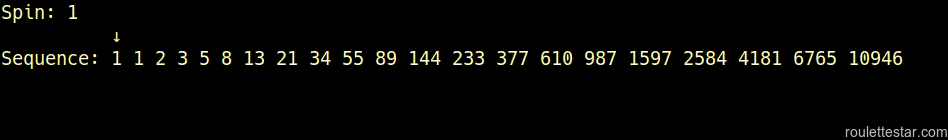The fibonacci system uses the fibonacci sequence of numbers to determine how much you should bet:

`1-1-2-3-5-8-13-21-34-55-89-144-233-377-610-987-1597-2584-4181-6765-10946-...`

The system is used when making even money wagers, such as betting on the outcome of red/black or even/odd on the roulette table.

You start at the number `1`, and move up and down the sequence depending on whether you won or lost on the last spin of the wheel.

• If you lose, you move one number up the sequence.
• If you win, you move two numbers down the sequence.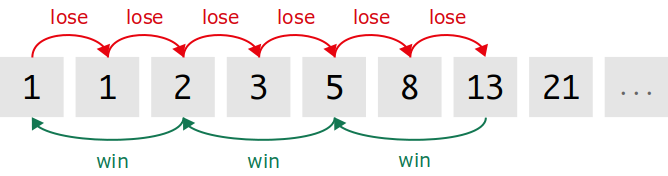The fibonacci sequence goes on forever, so you won't run out of numbers if you go on a long losing streak. Although the probability of losing on an evens bet (e.g. red) 20 times in a row is around 1 in 2,000,000, so it's unlikely that you'll need anything more than the first 20 fibonacci numbers.

Note: You just return to the start if you keep winning and cannot move any further back down the sequence.

## How do you use the fibonacci system?

Let's say you start with an initial bet of \$1. This is how you would use the fibonacci sequence:

• You bet `\$1`, and lose. So you move up the sequence.
• You bet `\$1`, and lose.
• You bet `\$2`, and lose.
• You bet `\$3`, and lose.
• You bet `\$5`, and win. So now you move two numbers down the sequence.
• You bet `\$2`, and lose.
• You bet `\$3`, and win.
• You bet `\$1`, and win. You now go back to the start.
• You bet `\$1`, ...

Basically you move up and down the fibonacci sequence depending on whether you won or lost your last bet. The only difficult part is keeping track of the sequence and knowing which number to move on to for calculating the size of your next bet.

It's similar to the martingale system because you increase the size of your bet when you are losing (i.e. a negative progression system). However, the increase is more gradual in the fibonacci system compared to the doubling in the martingale system, so your losses are less drastic when you hit a run of bad luck.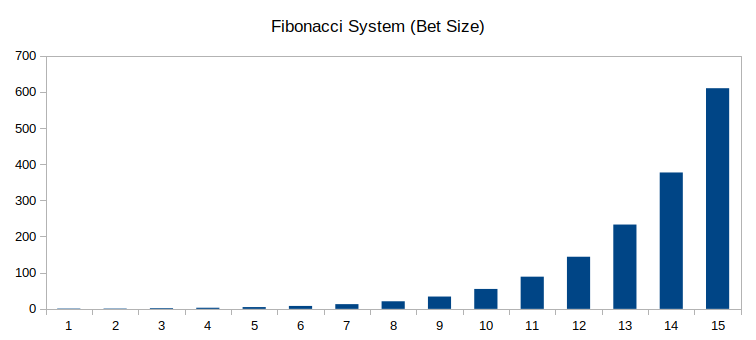The fibonacci system has a more gradual bet size progression, but it does end up increasing rapidly.

However, this does mean that it will take multiple spins to recoup your losses (as opposed to being able to recoup everything on your next spin like in the martingale system).

### Fibonacci sequence calculation

To work out how much you should be betting you just take your initial bet and multiply it by each number number in the sequence.

5
10
15
25
40
65
105
170
275
445
720
1165
1885
3050
4935
7985
12920
20905
33825
54730
...

## Fibonacci system simulation

This chart shows how your balance can fluctuate whilst using the fibonacci system during the course of a session.

Whilst it looks like your balance gradually increases over time, you occasionally run in to catastrophic losses that wipe out your bankroll. It's the same issue as in the martingale system

If you run a few simulations you'll see that some of the losses can be staggering. It's frightening to witness.

### Algorithm / Code

Here's some working Ruby code that shows how the fibonacci system works from a programming perspective. It works out (and stores) the next fibonacci number needed in the sequence as it runs.

``````# Settings
unit = 1
balance = 100
spins = 1000

# Initialize the fibonacci sequence (will work out the rest as we go)
fibonacci = [1, 1]

# Store our position in the fibonacci sequence
position = 0 # (zero is the first index in the sequence)

# Repeat for a given number of spins
spins.times do |i|

# Work out the bet size using the fibonacci sequence
bet = fibonacci[position] * unit

# Spin the roulette wheel
result = rand(0..36)

# Check if the result is a win
win = (result >= 19) # using the high numbers as the even-money wager

# Print bet size and result
print "Spin #{i}: bet=#{bet} #{win ? "(win)" : "(lose)"}, "

if win
# Update balance
balance = balance + bet

# Move back two positions in the sequence
position = position - 2

# Just go back to the start if we cannot go back two numbers
if position < 0
position = 0
end
else
# Update balance
balance = balance - bet

# Move forward one position in the sequence
position = position + 1

# Work out the next fibonacci number (if it's not already in the sequence)
if position > fibonacci.length - 1
fibonacci << fibonacci.last(2).inject(:+) # add last two numbers and append to the end
end
end

# Print new balance
print "balance=#{balance}\n"
end``````

## Where do the fibonacci numbers come from?

Each number in the sequence is the sum of the two previous numbers.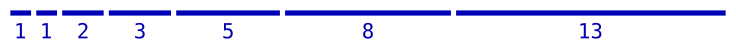So the next number after `8` and `13` will be `21`.

This simple sequence has surprisingly beautiful properties, and the numbers can be found within various branches of mathematics:The sequence can also be found in nature, and can be seen to describe things like:

• The spiral shape of a nautilus shell. (see below)
• The arrangement of seeds in a sunflower head.
• The structure of the scales on a pine cone.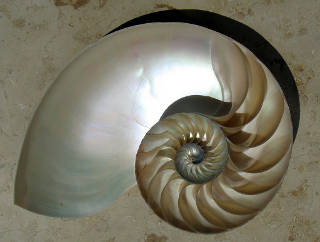So the fibonacci sequence of numbers may appear simple, but its structure carries special importance within both mathematics and the natural world.

So I guess these mysterious properties are the reason why the numbers have been as the basis of a betting system in the hope that they promote good fortune for some unknown reason.

### Who invented the fibonacci sequence?

I wouldn't say anyone invented the sequence, because it occurs in the natural world. But it was first introduced by the Italian mathematician Leonardo Bonacci in 1202.

His nickname was Fibonacci ("son of Bonacci").

However, whilst Fibonacci may have been the first to formally describe the sequence in a book, the sequence is reported to have been found as early as 200BC in India.

I'm not sure who the first person to use the sequence in roulette was though.

## Does the fibonacci system work?

Nope.

As interesting as the sequence is, there's nothing so special about it that it can overcome the house edge from one bet to the next.

I imagine the use of the system comes from some sort of belief in the divine power of the numbers. But whilst they may be a fundamental part of mathematics and the natural world, the sequence has no special ability to overcome the mathematics of the roulette wheel.

So don't be fooled by the mathematical origins of this system. It may be intriguing, but in reality it has no actual effect on the results of the roulette wheel.

Note: You can bet in any sequence you want, but it will never change the odds given to you by the roulette wheel.

## Conclusion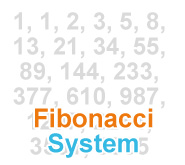The fibonacci system uses a fascinating sequence of numbers, but it has no effect on your ability to win money.

The use of the fibonacci system in roulette is like bashing on a rock with a rubber chicken. You might have the most mathematically beautiful chicken in the world, but at the end of the day, it's not going to change the underlying nature of the rock.*

Still, if nothing else, this particular system introduces you to the fibonacci numbers. Their connection with nature is pretty cool, and it's well worth exploring when you get bored of playing roulette.

* This was the best analogy I could think of.